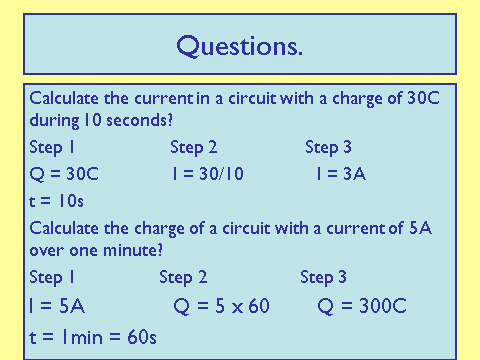# Electrical Charge and Current

## Electrical Current

Electric current is the flow of electric charge. The size of the current is the rate of flow of electrical charge. Electric charge is carried by electrons. In order for electric current to flow through a circuit, a source of potential difference (like a battery or a cell) must be included.

## Current Equation

You must be able to remember and apply this equation:

Charge flow = current x time

This can also be written as:Q = Charge (Coulombs, C)

I = Current (Amps, A)

t = Time (Seconds, s)

Around a single loop the current always remains the same.

Let’s look at some examples:Notice, in the second example, time is changed from minutes to seconds. Always use seconds!! Never minutes or hours.

Also, look at the three steps in showing ones working. You should always show your working, even if you use a calculator - and never forget to include units!

What is the unit of Charge?